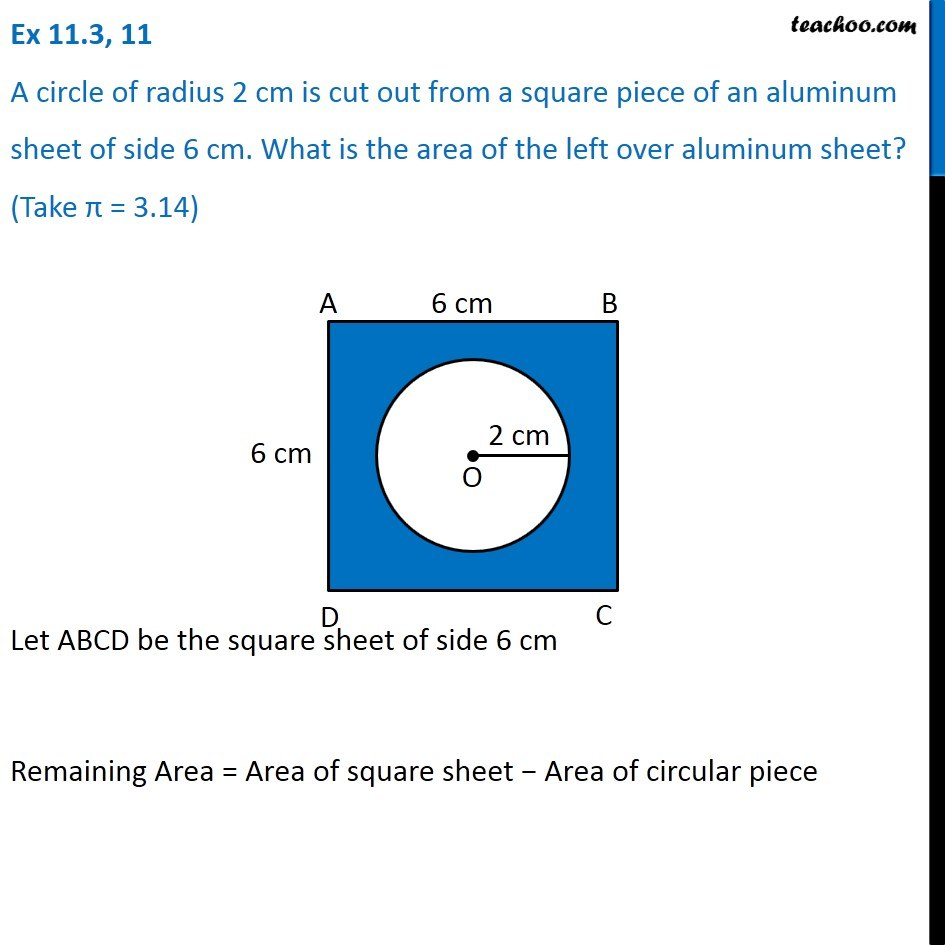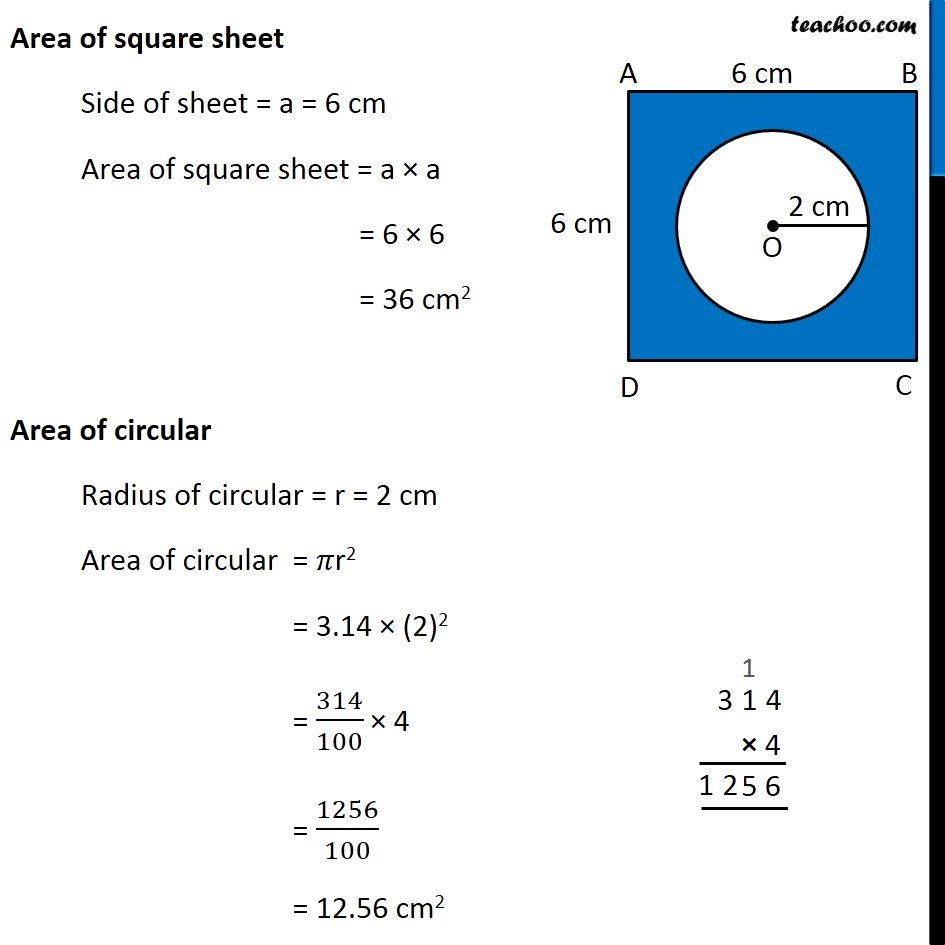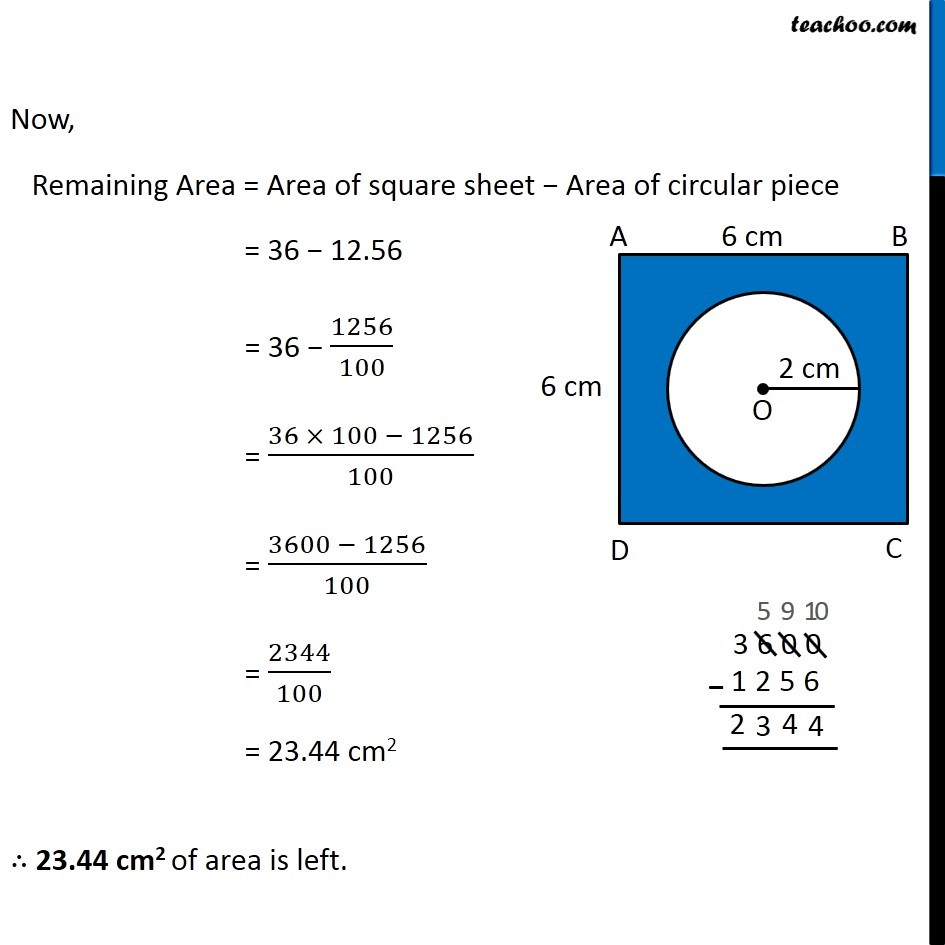Area of combination of figures - two circles, circle and square

Chapter 9 Class 7 Perimeter and Area
Concept wiseGet live Maths 1-on-1 Classs - Class 6 to 12

### Transcript

Ex 9.2, 11 A circle of radius 2 cm is cut out from a square piece of an aluminum sheet of side 6 cm. What is the area of the left over aluminum sheet? (Take π = 3.14) Let ABCD be the square sheet of side 6 cm Remaining Area = Area of square sheet − Area of circular piece Area of square sheet Side of sheet = a = 6 cm Area of square sheet = a × a = 6 × 6 = 36 cm2 Area of circular Radius of circular = r = 2 cm Area of circular = 𝜋r2 = 3.14 × (2)2 = 314/100 × 4 = 1256/100 = 12.56 cm2 Now, Remaining Area = Area of square sheet − Area of circular piece = 36 − 12.56 = 36 − 1256/100 = (36 × 100 − 1256)/100 = (3600 − 1256)/100 = 2344/100 = 23.44 cm2 ∴ 23.44 cm2 of area is left.